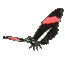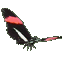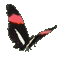Teaching Slope = Tangent Ratio     Trigonometry Fun     Math Help     Game Tips:

- The tangent of angle A can be written as tan A and equals the slope from point A(0,0) to point D(2,4).

This tangent ratio = slope = (rise)/(run) = (change in y)/(change in x) = (4-0)/(2-0) = (4)/(2) = 2/1.

- Generally, when angle A is in standard position as shown at this graph's origin (0,0)
then tan A equals the ratio = (rise)/(run) = slope.

- Recall, the slope between two points P(-2,5) and Q(4,-1) is (rise)/(run) = ((-1)-(5))/(4-(-2)) = (-6)/(6) = -1.
If the run = 0, then the vertical slope is said to be undefined.
- A slope of zero is a horizontal slope.
- The slope from a point P(x1,y1) to a point Q(x2,y2) = (change in y)/(change in x) = (y2-y1)/(x2-x1) .

- Your Game Score is reduced by the number of butterfly hits.

- To slow the game speed repeat tap/click on the word Slider.
- To increase the game speed repeat tap/click on the word Math.
- Speed can also be adjusted with a keyboard's - and + keys.

- Refresh/Reload the web page to restart the game.